## 15.3.1 Dubins Curves

Recall the Dubins version of the simple car given in Section 13.1.2. The system was specified in (13.15). It is assumed here that the car moves at constant forward speed,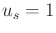. The other important constraint is the maximum steering angle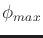, which results in a minimum turning radius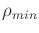. As the car travels, consider the length of the curve in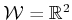traced out by a pencil attached to the center of the rear axle. This is the location of the body-frame origin in Figure 13.1. The task is to minimize the length of this curve as the car travels between any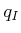and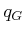. Due to, this can be considered as a bounded-curvature shortest-path problem. If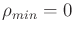, then there is no curvature bound, and the shortest path follows a straight line in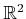. In terms of a cost functional of the form (8.39), the criterion to optimize is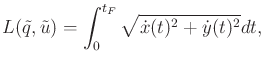(15.42)

in which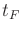is the time at whichis reached, and a configuration is denoted as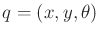. Ifis not reached, then it is assumed that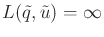.

Since the speed is constant, the system can be simplified to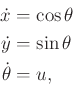(15.43)

in whichis chosen from the interval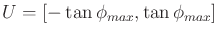. This implies that (15.42) reduces to optimizing the timeto reachbecause the integrand reduces to. For simplicity, assume that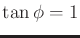. The following results also hold for any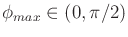.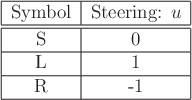It was shown in  that between any two configurations, the shortest path for the Dubins car can always be expressed as a combination of no more than three motion primitives. Each motion primitive applies a constant action over an interval of time. Furthermore, the only actions that are needed to traverse the shortest paths are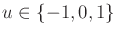. The primitives and their associated symbols are shown in Figure 15.3. The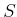primitive drives the car straight ahead. The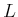and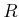primitives turn as sharply as possible to the left and right, respectively. Using these symbols, each possible kind of shortest path can be designated as a sequence of three symbols that corresponds to the order in which the primitives are applied. Let such a sequence be called a word . There is no need to have two consecutive primitives of the same kind because they can be merged into one. Under this observation, ten possible words of length three are possible. Dubins showed that only these six words are possibly optimal: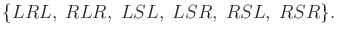(15.44)

The shortest path between any two configurations can always be characterized by one of these words. These are called the Dubins curves.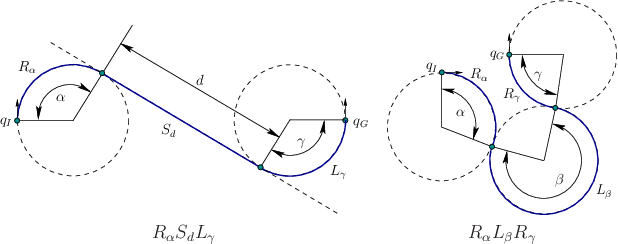To be more precise, the duration of each primitive should also be specified. Foror, let a subscript denote the total amount of rotation that accumulates during the application of the primitive. For, let a subscript denote the total distance traveled. Using such subscripts, the Dubins curves can be more precisely characterized as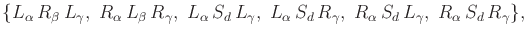(15.45)

in which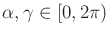,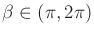, and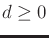. Figure 15.4 illustrates two cases. Note that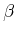must be greater than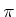(if it is less, then some other word becomes optimal).

It will be convenient to invent a compressed form of the words to group together paths that are qualitatively similar. This will be particularly valuable when Reeds-Shepp curves are introduced in Section 15.3.2 because there are 46 of them, as opposed to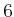Dubins curves. Let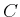denote a symbol that means curve,'' and represents eitheror. Using, the six words in (15.44) can be compressed to only two base words: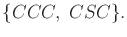(15.46)

In this compressed form, remember that two consecutives must be filled in by distinct turns (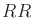and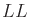are not allowed as subsequences). In compressed form, the base words can be specified more precisely as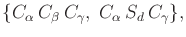(15.47)

in which,, and.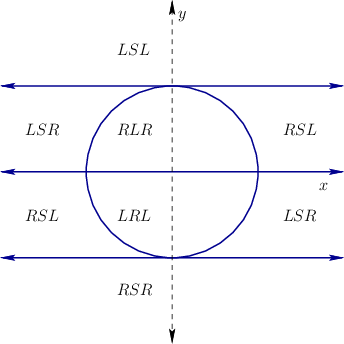Powerful information has been provided so far for characterizing the shortest paths; however, for a givenand, two problems remain:

1. Which of the six words in (15.45) yields the shortest path betweenand?
2. What are the values of the subscripts,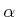,,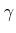, and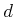for the particular word?
To use the Dubins curves as an LPM, these questions should be answered efficiently. One simple approach is to try all six words and choose the shortest one. The parameters for each word can be determined by tracing out minimum-radius circles fromand, as shown in Figure 14.23. Another way is to use the precise characterization of the regions over which a particular word is optimal. Suppose thatis fixed at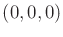. Based on the possible placements of, the C-space can be partitioned into cells for which the same word is optimal. The cells and their boundaries are given precisely in . As an example, a slice of the cell decomposition for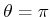is shown in Figure 15.5.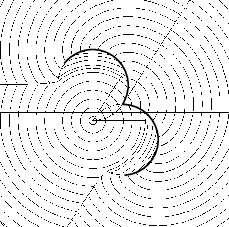In addition to use as an LPM, the resulting cost of the shortest path may be a useful distance function in many sampling-based planning algorithms. This is sometimes called the Dubins metric (it is not, however, a true metric because it violates the symmetry axiom). This can be considered as the optimal cost-to-go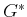. It could have been computed approximately using the dynamic programming approach in Section 14.5; however, thanks to careful analysis, the exact values are known. One interesting property of the Dubins metric is that it is discontinuous; see Figure 15.6. Compare the cost of traveling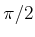using theprimitive to the cost of traveling to a nearby point that would require a smaller turning radius than that achieved by theprimitive. The required action does not exist in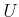, and the point will have to be reached by a longer sequence of primitives. The discontinuity inis enabled by the fact that the Dubins car fails to possess the STLC property from Section 15.1.3. For STLC systems,is continuous.

Steven M LaValle 2020-08-14